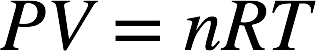# Problem: Calculate the density of Freon 12, CF2Cl2, at 30.0 °C and 0.954 atm

###### FREE Expert Solution

The ideal gas law is as follows:Recall that the number of moles (n) is given by:where mass = the mass of the gas in grams and M = molar mass of the gas in g/mol. We can plug this equation into the ideal gas law:We can rearrange this equation to have mass/V on one side. Note that mass/V is actually density (d):This will be our working equation for the problem.

89% (21 ratings)###### Problem Details

Calculate the density of Freon 12, CF2Cl2, at 30.0 °C and 0.954 atm